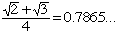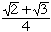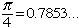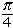Name: Tania Who is asking: Parent Level: All Question: I really need your help... How could I show (and explain to my son) that any countably infinite set has uncontably many infinite subsets of which any two have only a finite number of elements in common? Thanks, Tania Hi Tania, One nice proof comes from the fact that the interval (0,1) is uncountable, while the set of terminating fractions between 0 and 1 is countable. This means that to every real number in (0,1), you can associate the set of its approximations in terminating fractions (without rounding up):so you would associate tothe set {0.7, 0.78, 0.786, 0.7865, ...}, so you would associate tothe set {0.7, 0.78, 0.785, 0.7853, ...} In this way you get an uncountable family of sets of rational numbers. Any two number in (0,1) can have the same decimal expansion only up to a certain (finite) decimal, which means that the corresponding sets only have a finite number of elements (that is, approximations) in common. Claude Go to Math Central